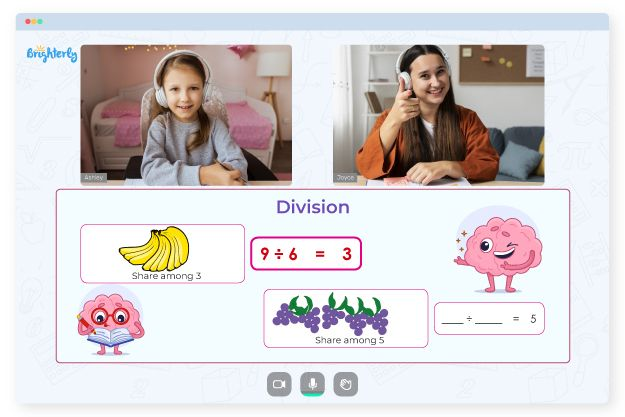# Basic Dividing Worksheets

Basic division is a foundational skill that kids have to master to move on to more complex math concepts. By using basic dividing worksheets, your youngster can apply what they have learned about addition, subtraction, and multiplication to start mastering division. With time, they can proceed to learning long division with multi-digit numbers and remainders.

Keep reading to know more about basic dividing worksheets for students learning division.

## Why Tutors from Brighterly Use Basic Dividing Worksheets

The tutors from Brighterly use basic diving worksheets to help students have a better shot at understanding division. Brighterly is an online math platform with professionals who use basic division worksheets and games to help kids enjoy math. Once students have learned multiplication tables, they can understand how to divide using a divisor of just one number. The worksheets come with time tables behind so kids can learn multiplication facts before learning more complex math concepts.

Math for Kids

Is Your Child Struggling With Math?
1:1 Online Math TutoringStudents will also use the chunking strategy to repeatedly subtract the divisor from the dividend until they get the correct answer. The simple division worksheets serve as the foundational aspect of learning where kids get all they would need for building their division skills.

## How to Use Basic Dividing Worksheets in a Fun Way

Tutors use easy division worksheets to divide a big number by a smaller one. Professionals design worksheets for kids to have fun and enjoy studying.### Basic Dividing Worksheets PDF

Simple Division Worksheet### Basic Dividing Worksheets PDF

Simple Division Worksheets### Basic Dividing Worksheets PDF

Basic Division Worksheets### Basic Dividing Worksheets PDF

Easy Division Worksheets

With time, using a basic division worksheet, kids will understand that math is not a challenging subject but can also be fun. There are also exciting exercises and activities in the simple division worksheet that can be used by kids to have a great time.

### More Division Worksheets

Problems with Division?• Is your child having trouble with mastering division concepts?
• Start learning with an online tutor.

Does your child need extra support in understanding division concepts? An online tutor could help.